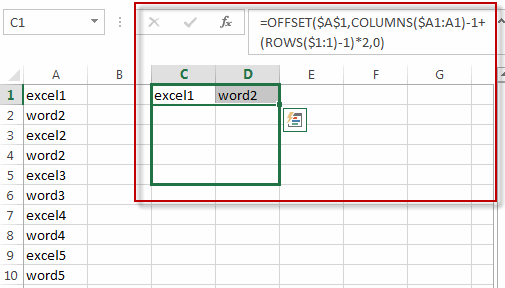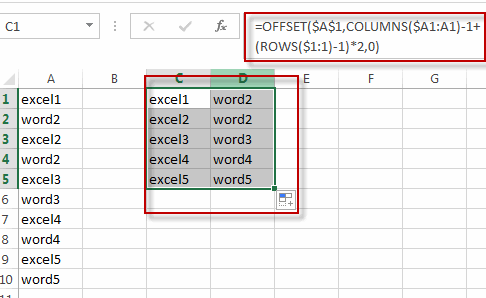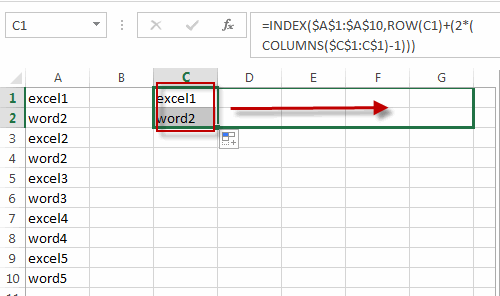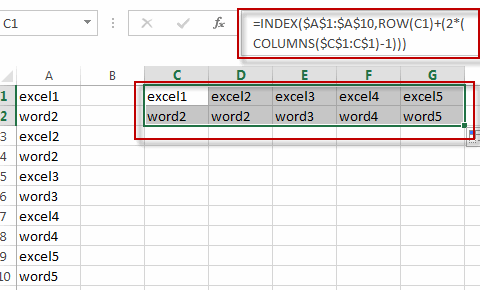# Split Data in One Column to Multiple Columns

This post will guide you how to split data in a single column into multiple columns with a formula in Excel. How do I transpose data from one column to multiple columns in Excel. How can I make one long column into multiple columns in Excel.

## Split Data in One Column to Multiple Columns

Assuming that you have 5 sets of product’s list in one column, and you would like to convert each set in its own column or split each sets into its own staked columns. How to achieve it.

If you want to split each sets in one column from row to row in stacked columns, and you need to create a formula based on the OFFSET function, the COLUMNS function, and the ROWS function.

Just like this:

`=OFFSET(\$A\$1,COLUMNS(\$A1:A1)-1+(ROWS(\$1:1)-1)*2,0)`

Type this formula into a blank cell C1, and press Enter key in your keyboard, and then drag the AutoFill Handle from C1 to D1. Select the Cell C1 and D1, then drag the AutoFill Handle in Cell D1 from D1 to D5.If you want to split each sets in one column from one column into another column, you just need to use the following formula based on the INDEX function, the ROW function and the COLUMNS function. Like this:

`=INDEX(\$A\$1:\$A\$10,ROW(C1)+(2*(COLUMNS(\$C\$1:C\$1)-1)))`

Type this formula into the formula box of the Cell C1, and press Enter key in your keyboard, and drag the AutoFill Handle from C1 to C2. Select the Cell C1 and C2, and then drag the AutoFill handle in Cell C2 from C2 to G2.### Related Functions

• Excel INDEX function
The Excel INDEX function returns a value from a table based on the index (row number and column number)The INDEX function is a build-in function in Microsoft Excel and it is categorized as a Lookup and Reference Function.The syntax of the INDEX function is as below:= INDEX (array, row_num,[column_num])…
• Excel ROW function
The Excel ROW function returns the row number of a cell reference.The ROW function is a build-in function in Microsoft Excel and it is categorized as a Lookup and Reference Function.The syntax of the ROW function is as below:= ROW ([reference])….
• Excel ROWS function
The Excel ROWS function returns the number of rows in a cell reference.The ROWS function is a build-in function in Microsoft Excel and it is categorized as a Lookup and Reference Function.The syntax of the ROWS function is as below:= ROWS(array)….
• Excel COLUMNS function
The Excel COLUMNS function returns the number of columns in an Array or a reference.The syntax of the COLUMNS function is as below:=COLUMNS (array)….